﻿ A Fast Fractional-Pixel Search Algorithm Based on Linear-Prediction Motion EstimationAmerican Journal of Signal Processing

p-ISSN: 2165-9354    e-ISSN: 2165-9362

2018;  8(1): 20-26

doi:10.5923/j.ajsp.20180801.03### A Fast Fractional-Pixel Search Algorithm Based on Linear-Prediction Motion Estimation

Lung-Jen Wang, Wen-Ming Tai

Dept. of Computer Science and Information Engineering, National Pingtung University, Taiwan, R.O.C.

Correspondence to: Lung-Jen Wang, Dept. of Computer Science and Information Engineering, National Pingtung University, Taiwan, R.O.C..
 Email: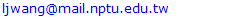Abstract

The fractional-pixel motion estimation is used accurately for motion vector prediction in the H.264/AVC video coding. Based on the linear prediction and a small diamond search algorithm, a fast fractional-pixel search algorithm is proposed in this paper. The proposed method substantially solves the complexity of the calculation of the fractional-pixel motion estimation needed in the H.264/AVC video coding if the image resolution is increased. Finally, experimental results show that the proposed algorithm is superior in performance and reduces around 60% computations for the fractional-pixel calculations.

Keywords: Fractional-pixel, Motion estimation, Linear prediction, H.264/AVC

Cite this paper: Lung-Jen Wang, Wen-Ming Tai, A Fast Fractional-Pixel Search Algorithm Based on Linear-Prediction Motion Estimation, American Journal of Signal Processing, Vol. 8 No. 1, 2018, pp. 20-26. doi: 10.5923/j.ajsp.20180801.03.

### 1. Introduction

The H.264/AVC algorithm is one of the international standard of video coding technique [1, 2]. To improve the accuracy of motion estimation in the H.264/AVC video coding, a fractional-pixel motion estimation algorithm is almost mandatory [3, 4]. In addition, it is used the interpolation process to estimate the fractional pixel (1/2-pel and 1/4-pel) positions between the existing positions for the motion vector prediction in the reference image which is magnified in image resolution. In the typical hierarchical fractional-pixel search (HFPS) algorithm  shown in Fig.1, at least 8 positions are required for the 1/2-pel, and 16 positions are required for the 1/4-pel, respectively. The disadvantage of the 1/2-pel and 1/4-pel fractional pixel search algorithm is that the computations required are becoming very large. Furthermore, if the image resolution is increased, there are needed for additional motion estimation computations that involve considerably more fractional pixels.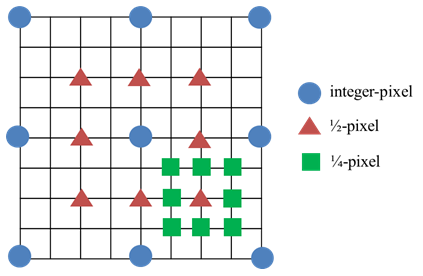Figure 1. The hierarchical fractional-pixel search (HFPS) algorithm
Many fast fractional-pixel motion estimation algorithms to reduce the computational complexity of the motion estimation search process have been proposed [3, 4, 6-9] [12-16] such as Center Biased Fractional Pixel Search (CBFPS) , Fast Fractional Pixel Search (FFPS) , and Linear Prediction Search (LPS) . The authors in  also developed a fast fractional-pixel search (FFS) algorithm to solve the calculation of the fractional-pixel motion estimation needed in the H.264/ AVC algorithm. In this paper, the FFS algorithm based on linear-prediction motion estimation, called LFFS method, is proposed. That is, the more detailed description and derivation of LFFS method are presented in this paper. In addition, this LFFS method uses the linear prediction and small diamond search algorithm, as shown in Fig.2, [3, 6, 9]. It substantially reduces the fractional-pixel computations that are based on the sum of absolute difference error surface. Furthermore, computer simulations show that the proposed method speeds up the H.264/AVC standard and still achieves a very good quality of reconstructed image for motion vector prediction.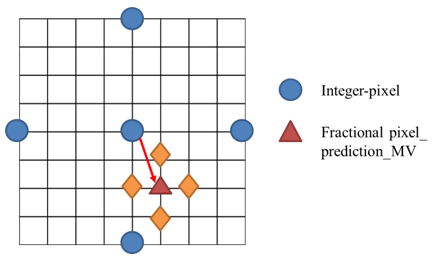Figure 2. The small diamond search algorithm

### 2. Fractional-Pixel Motion Estimation

The fractional-pixel motion estimation of the H.264/AVC video coding can be used to improve the inter-frame prediction for video quality . It requires very large computations, such as fractional-pixel (1/2-pel and 1/4-pel) search algorithm and interpolation process. Furthermore, the displacement vector with fractional-pixel resolution can be applied for the motion vector prediction in the H.264/AVC algorithm . To estimate the fractional-pixel displacement, a two-step interpolation process is used as shown in Fig. 3. In the H.264/AVC algorithm, the Wiener filter with six coefficients: [1, -5, 20, 20, -5, 1]/32 is used to interpolate the 1/2-pel positions in first step and an image IW is generated after the first interpolation step. In addition, the bilinear interpolation filter is used to interpolate the 1/4-pel positions and an image IB is generated after the second interpolation step. Fig. 4 shows the interpolation relationship between the integer-pel positions (black), the 1/2-pel positions (gray) and the 1/4-pel positions (white) .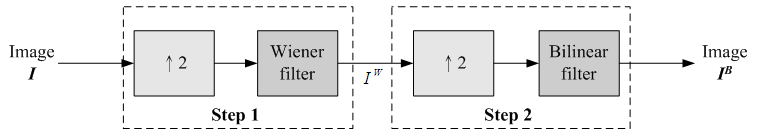Figure 3. Two-step interpolation process used in H.264/AVC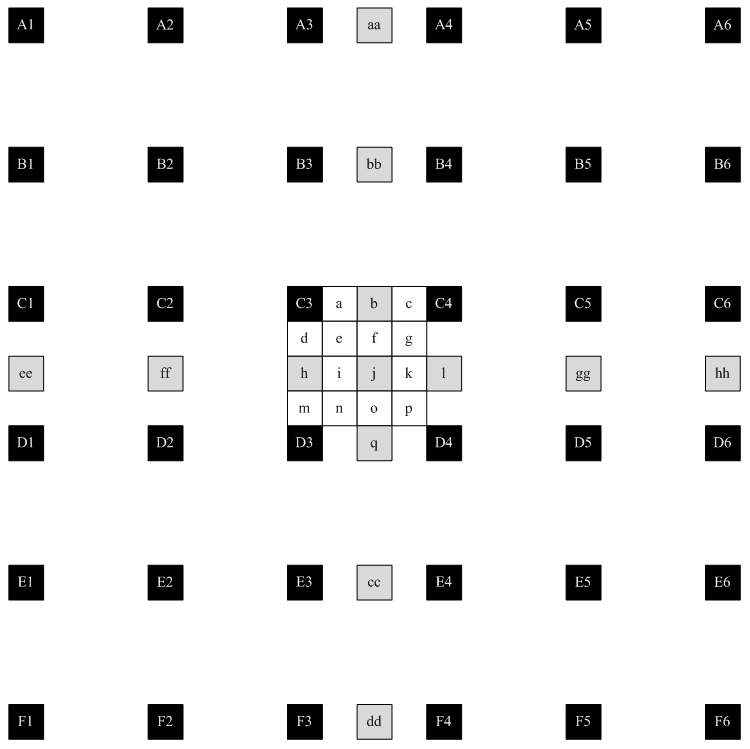Figure 4. Example of interpolation relationship for filter size 6×6
In the H.264/AVC algorithm, each image is divided into macroblocks of size N×N. By default, N=16 for luminance images. For chrominance images, N=8 if 4:2:0 chroma subsampling is adopted. The current image frame is referred to as target frame. A match is sought between the macroblock in the target frame and the most similar macroblock in previous and/or future frame(s) (referred to as reference frame(s)). The displacement of the reference macroblock to the target macroblock is called a motion vector MV. The difference between two macroblocks can then be measured by their sum of absolute difference (SAD):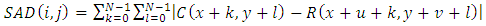(1)
where N is size of the macroblock, k and l are indices for pixels in the macroblock, i and j are horizontal and vertical displacements, C(x+k,y+l) is pixels in macroblock in target frame, R(x+u+k,y+v+l) is pixels in macroblock in reference frame and the motion vector MV=(u,v) such that SAD(i, j) is minimum. In addition, one observation is that if the minimum SAD obtained at fractional-pixel accuracy is larger than that at integer-pixel accuracy, the motion vector on the integer-pixel is selected as the final result and the fractional-pixel search for this block is regarded as ineffective. Otherwise, the fractional-pixel search is regarded as effective and the motion vector on the fractional-pixel is selected as the final result [8, 9].
Table 1 shows the effective fractional-pixel search ratio for some test video sequences. For the sequences with relatively lower motions, such as Paris with 38% and Akiyo with 48%, the majority of the fractional-pixel search is ineffective. The ineffective fractional-pixel search ratios are 62% and 52% for Paris and Akiyo, respectively. That is, the integer-pixel search is enough for the sequences with lower motions. Obviously, in Table 1, the most of fractional-pixel searches is ineffective. Therefore, if the integer-pixel motion vector is selected as the final motion vector, the fractional-pixel search computations can be skipped. In this paper, the LFFS method is proposed to determine whether the fractional-pixel search should be performed or not [8, 9].
 Table 1. Effective Fractional-pixel Searsh Ratio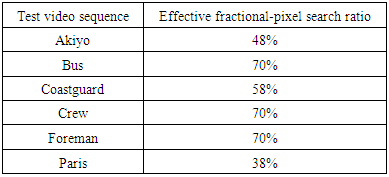### 3. The Proposed LFFS Algorithm

#### 3.1. Linear Prediction

In this paper, a linear prediction based the fractional -pixel motion estimation and the center-biased fractional-pixel search (CBFPS)  is developed, first estimates horizontal components and next vertical components for the fractional-pixel motion vector and its sum of absolute difference error surface. Figs. 5 and 6 show the linear prediction of fractional-pixel error surface in the horizontal and vertical directions, respectively.
In Fig. 5 for the x-axis (horizontal direction), letand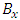be integer-pixel points, and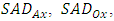and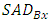are their corresponding SADs.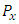is selected point between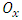and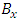points such that the slopes of two lines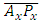and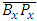are equal as follows.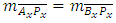(2)
Let s be the distance between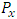in a predicted minimum point andin a fractional-pixel point, and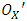be a point in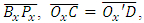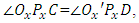and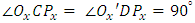. The distance s can be obtained by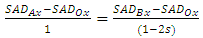(3)
and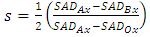(4)
Following , if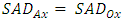, let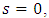i.e., the integer-pixel motion vector is selected as the final motion vector.
Similarly, let t be selected distance between a predicted minimum point and a fractional-pixel point in the y-axis (vertical direction) for the fractional-pixel motion vector. As shown in Fig. 6, the distance t can be given by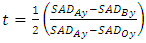(5)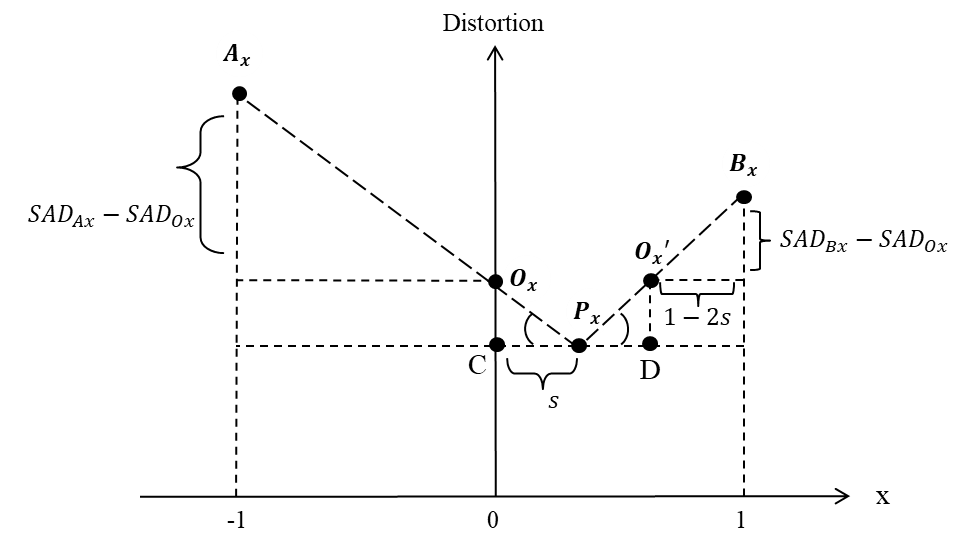Figure 5. A linear prediction fractional-pixel error surface in x-axis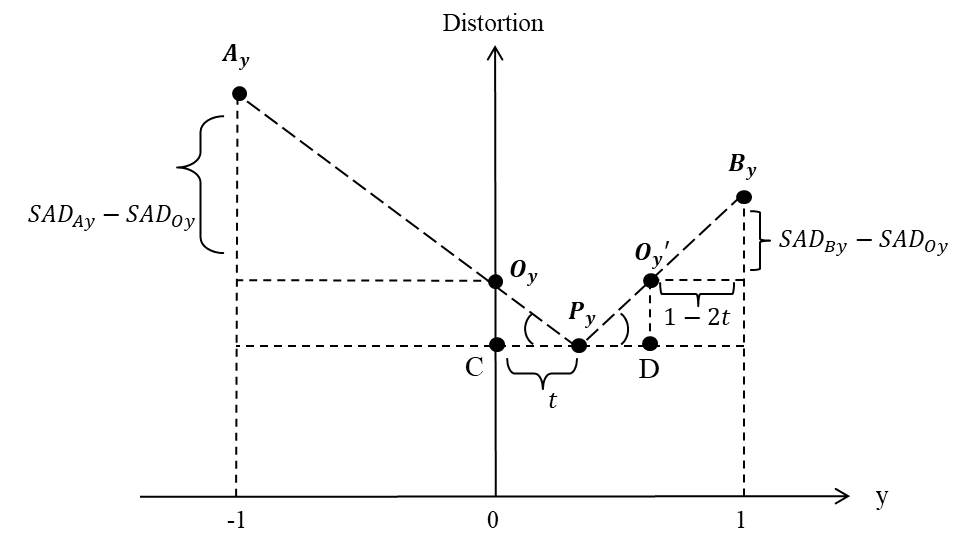Figure 6. A linear prediction fractional-pixel error surface in y-axis

#### 3.2. LFFS with a Small Diamond Search

In this paper, the LFFS algorithm combines (4) and (5) as the fractional-pixel search direction as follows.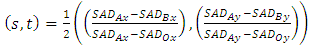(6)
Using (6), we can calculate the s and t values at the best integer-pixel position and determinate the fractional-pixel search direction according to a small diamond search with their corresponding s and t values.
Furthermore, some directional selections based on the small diamond search algorithm are developed as shown in Fig. 7. The detailed flow chart of the proposed LFFS algorithm is mentioned in Fig. 8. In addition, Table 2 shows the directional selection of the fractional-pixel search.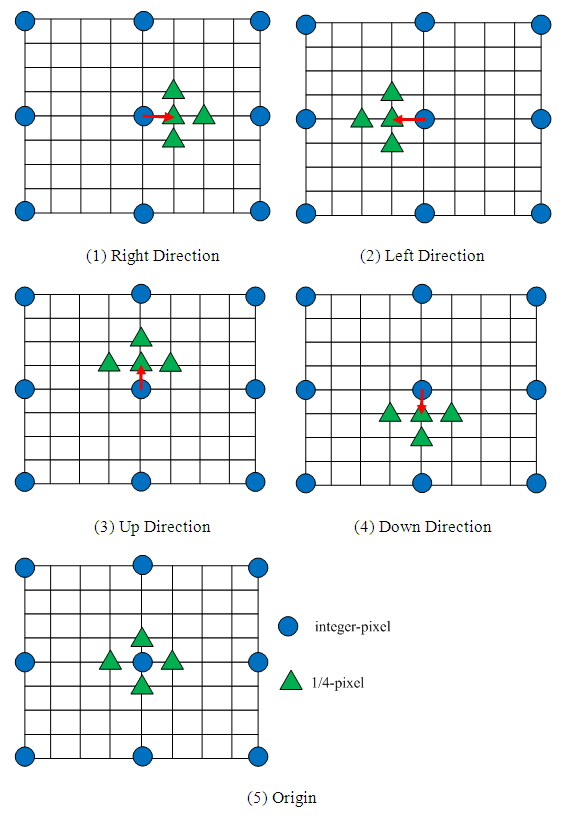Figure 7. Directional selections based small diamond search
 Table 2. Directional Selections for Fractional-Pixel Search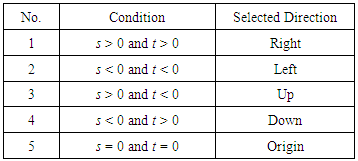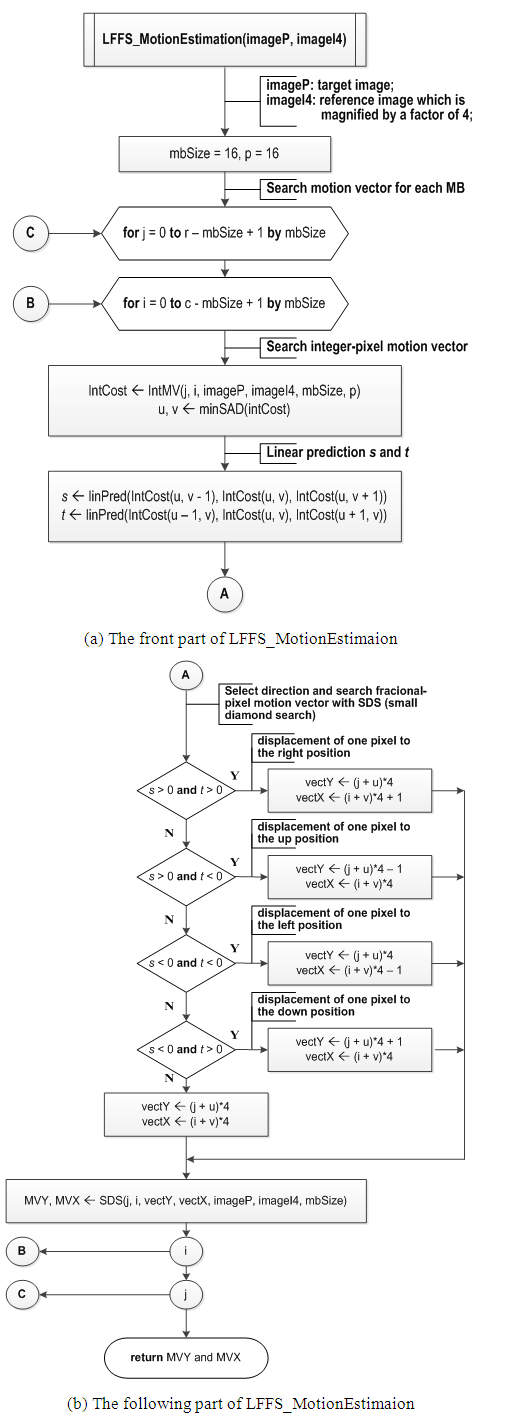Figure 8. The flow chart of the proposed LFFS algorithm

### 4. Experimental Results

In this paper, the proposed LFFS method is compared with the Hierarchical Fractional Pixel Search (HFPS) , the Center Biased Fractional Pixel Search (CBFPS) , the Fast Fractional Pixel Search (FFPS) , and the Linear Prediction Search (LPS) . Performance comparisons are carried out on some standard CIF (Akiyo, Bus, Coastguard, Crew, Foreman, Paris) and 4CIF (City, Harbour, Ice, Soccer) video sequences shown in Figs. 9 and 10, and based on the H.264/AVC JM 18.0 .Figure 9. Some standard video test sequences (CIF)Figure 10. Some standard video test sequences (4CIF)
Experimental setups are IPPPP… frame structure, 1 reference frame, four different QP values (28, 32, 36, and 40), ±16 search range, and 100 frames. All the reported results (bit rates (kbps), number of search points (#SP), and PSNR (dB) performance) are computed from the reconstructed Y components.
Table 3 shows the performance comparisons for Akiyo (CIF) video sequences. In this table:
(1) At QP=28, for the ΔPSNR which is compared to the HFPS method, the proposed LFFS method is -0.01dB (least loss); for the average number of search points (#SP), the LPS method is 5.35 which is less than the other methods; and for bit-rates, the HFPS method is 443.47kbps and less than the other methods.
(2) At QP=32, for the ΔPSNR, the LPS method is -0.01dB (least loss); for the average number of search points (#SP) and bit-rates, the LPS method is 5.49 and 313.26kbps, respectively, which is also less than the other methods.
(3) At QP=36, for the ΔPSNR, the proposed LFFS method is 0dB (no loss); for the average number of search points (#SP), the LPS method is 5.64 which is less than the other methods; and for bit-rates, the CBFPS method is 245.37kbps and less than the other methods.
(4) At QP=40, for the ΔPSNR, the proposed LFFS method is 0dB (no loss) and the CBFPS and LPS methods are +0.03dB which are better than HFPS method; for the average number of search points (#SP), the LPS method is 5.77 which is less than the other methods; and for bit-rates, the HFPS method is 216.38kbps and less than the other methods.
 Table 3. Performance Comparisons for Akiyo (CIF)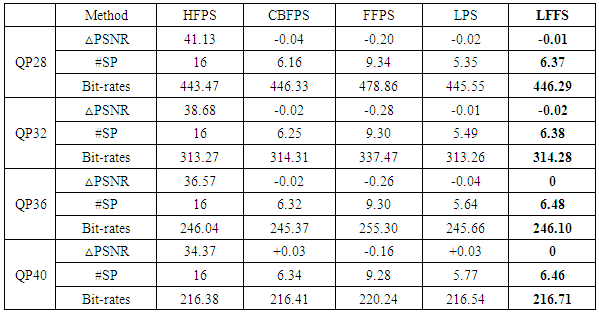In addition, Table 4 shows the performance comparisons for Ice (4CIF) video sequences. In this table:
(1) At QP=28, for the ΔPSNR which is compared to the HFPS method, the proposed LFFS method and CBFPS method are 0dB (no loss); for the average number of search points (#SP) and bit-rates, the proposed LFFS method is 7.65 and 2737.14kbps, respectively, which is less than the other methods.
(2) At QP=32, for the ΔPSNR, the CBFPS method is +0.02dB and the proposed LFFS method is +0.01dB which are better than HFPS method; for the average number of search points (#SP), the proposed LFFS method is 7.70 which is less than the other methods; and for bit-rates, the CBFPS method is 1834.02kbps and less than the other methods.
(3) At QP=36, for the ΔPSNR, the proposed LFFS method is +0.02dB which is better than HFPS and other methods; for the average number of search points (#SP) and bit-rates, the proposed LFFS method is 7.65 and 1329.52kbps, respectively, which is also less than the other methods.
(4) At QP=40, for the ΔPSNR, the proposed LFFS method is +0.01dB which is better than HFPS and other methods; for the average number of search points (#SP) and bit-rates, the proposed LFFS method is 7.56 and 1068.10kbps, respectively, which is also less than the other methods.
 Table 4. Performance Comparisons for Ice (4CIF)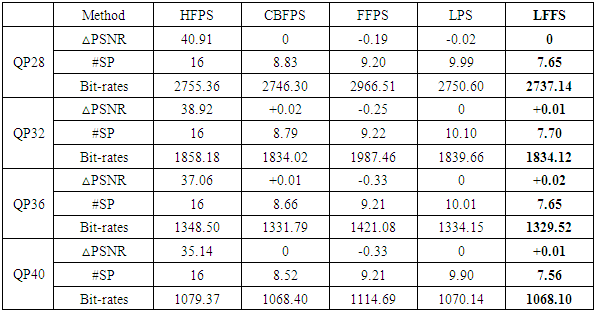Finally, based on the above computer simulations, we can summarize the experimental results as follows:
(1) For the above standard CIF (Akiyo, Bus, Coastguard, Crew, Foreman, Paris) video sequences:
(a) The proposed LFFS method is compared with the HFPS method, the ΔPSNR is -0.08dB (most loss), and however, for the average number of search points (#SP), the proposed LFFS method is less 60% than the HFPS method.
(b) The proposed LFFS method is compared with the CBFPS method, the ΔPSNR is similar, and for the average number of search points (#SP), the proposed LFFS method is less 29% than the CBFPS method.
(c) The proposed LFFS method is compared with the FFPS method, the ΔPSNR is more than 0.3dB, and for the average number of search points (#SP), the proposed LFFS method is less 31% than the FFPS method.
(d) The proposed LFFS method is compared with the LPS method, the ΔPSNR is more than 0.01dB than the LPS method, and for the average number of search points (#SP), the proposed LFFS method is less 30% than the LPS method.
(2) For the above standard 4CIF (City, Harbour, Ice, Soccer) video sequences:
(a) The proposed LFFS method is compared with the HFPS, CBFPS, and LPS methods, the ΔPSNR is similar, and for the average number of search points (#SP), the proposed LFFS method is less 52% than these methods.
(b) The proposed LFFS method is compared with the FFPS method, the ΔPSNR is more than 0.37dB than the FFPS method, and for the average number of search points (#SP), the proposed LFFS method is less 16% than the FFPS method.

### 5. Conclusions

In the H.264/AVC standard, the typical HFPS algorithm is used to improve the fractional-pixel motion estimation. This HFPS method requires at least 8 positions for the 1/2-pel, and 16 positions for the 1/4-pel, respectively, in the fractional-pixel motion estimation. However, if the image resolution is increased, the computations required for the fractional-pixel motion estimation are mandatory increased. In order to reduce the additional calculations of the fractional-pixel motion estimation needed in the H.264/AVC algorithm, the linear-prediction fast fractional-pixel search algorithm, called LFFS method, is developed in this paper. In this method, both linear prediction and small diamond search are proposed. Furthermore, the proposed LFFS method substantially reduces 60% and 52% computations for the fractional-pixel motion estimation in CIF and 4CIF video sequences, respectively, and still achieves a better quality of reconstructed image. That is, the proposed method for the fractional-pixel motion estimation in the H.264/AVC standard is that it substantially reduces the computation complexity and also increases the precision of motion vector prediction.

### ACKNOWLEDGEMENTS

This work was supported by the Ministry of Science and Technology, R.O.C., under Grant MOST 104-2221-E-153-010, 106-2622-E-153-001-CC2 and 106-2221-E-153-006.

### References

  T. Wiegand, G. J. Sullivan, G. Bjontegaard, and A. Luthra, “Overview of the H.264/AVC video coding standard,” IEEE Trans. on Circuits and Systems for Video Technology, vol. 13, no. 7, pp. 560-576, July 2003.  T. Wiegand and G. J. Sullivan, “The H.264/AVC video coding standard [Standards in a Nutshell],” IEEE Signal Processing Magazine, vol.24, no.2, pp.148-153, March 2007.  Z. Chen, P. Zhou, and Y. He, “Fast integer pel and fractional pel motion estimation for JVT,” JVT-F017, Awaji Japan, Dec. 2002.  Z. Wei and Z. Xin, “A fast hierarchical 1/4-pel fractional pixel motion estimation algorithm of H.264/AVC video coding,” in Proc. 8th IEEE Conference on Industrial Electronics and Applications, pp. 891-895, 2013.  L. J. Wang and W. M. Tai, “A fast linear-prediction fractional-pixel search algorithm,” in Proc. of the 30th IEEE International Conference on Advanced Information Networking and Applications (IEEE AINA-2016), Le Régent Congress Centre, Crans-Montana, Switzerland, March 23-25, 2016.  H. Nisar and T. S. Choi, “Fast and efficient fractional pixel motion estimation for H.264/AVC video coding,” in Proc. 16th IEEE International Conference on Image Processing, pp. 1561-1564, 2009.  K. H. Ng, L. M. Po, S. Y. Li, K. M. Wong and L. P. Wang, “A Linear prediction based fractional-pixel motion estimation algorithm,” in Proc. 4th International Conference on Multimedia and Ubiquitous Engineering, pp. 1-6, 2010.  J. S. Kim, K. W. Lee and M. H. Sunwoo, “Novel fractional pixel motion estimation algorithm using motion prediction and fast search pattern,” in Proc. IEEE International Conference on Multimedia and Expo, pp.821-824, 2008.  L. Shen, Z. Zhang, Z. Liu and W. Zhang, “An adaptive fractional pixel search algorithm,” in Proc. Fourth International Conference on Intelligent Sensing and Information Processing, pp. 153-156, 2006.  L. J. Wang and C. T. Shu, “An efficient fractional-pixel motion compensation based on cubic convolution interpolation,” Journal of Electrical and Electronic Engineering, vol. 2, no. 4, pp. 52-59, Sept. 2014.  H.264/AVC Reference Software Version JM18.0, [Online]. Available: http://iphome.hhi.de/suehring/tml/download/old_jm/.  J. F. Chang and J. J. Leou, “A quadratic prediction based fractional-pixel motion estimation algorithm for H.264,” in Proc. Seventh IEEE International Symposium on Multimedia, 2005.  J. Fang, W. Zheng, D. Zhang, and K. Wang, “A mode correlation-based fractional pixel motion estimation for H.264 video coding,” in Proc. 7th International Conference on ASIC, pp. 938- 941, 2007.  S. G. Deshpande and J. N. Hwang, “A new fast motion estimation method based on total least squares for video encoding,” in Proc. IEEE International Conference on Acoustics, Speech and Signal Processing, pp. 2797-2800, 1998.  R. Husemann, V. Roesler, R. Kintschner, H. Fröhlich, and A. A. Susin, “High performance H.264/AVC encoding motion prediction algorithm,” in Proc. 18th IEEE International Conference on Image Processing, pp. 957-960, 2011.  T. S. Kim, C. E. Rhee, H. J. Lee, and S. I. Chae, “Fast integer motion estimation with bottom-up motion vector prediction for an HEVC encoder,” IEEE Transactions on Circuits and Systems for Video Technology, 2017.